11月16日，2018广州国际汽车展览会盛大开幕。哈弗品牌以“冠军哈弗 智联新时代”为主题，携旗下H、F多款重磅车型亮相，双系并立惊艳全场。其中，全新哈弗H6冠军版及哈弗H4智联版正式上市，标志着哈弗品牌全面进入智联新时代。同时，哈弗F系近期相继上市的F5、F7，以及H系哈弗H2、哈弗H6Coupe、哈弗H7、哈弗H9等实力车型也悉数亮相，给广大参观者带来了极致的视觉冲击和观车体验。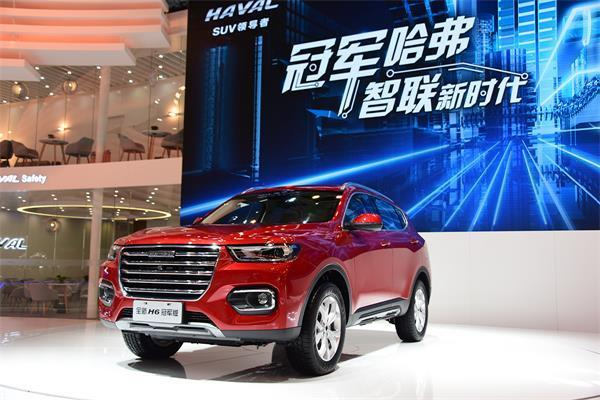开启智联新时代  H系利剑再出鞘

作为哈弗品牌在本次车展中的重头戏，全新哈弗H6冠军版及哈弗H4智联版一经上市，便吸引众多媒体及参观者驻足，赚足眼球。其中，全新哈弗H6共上市包括冠军版等7款车型，分别搭载1.5GDIT和2.0GDIT两款动力，售价区间为10.2-13.4万元。哈弗H4智联版定位“8万级高能智联SUV”，有手动、自动挡共计6款车型可选，售价区间为7.9-11.3万元。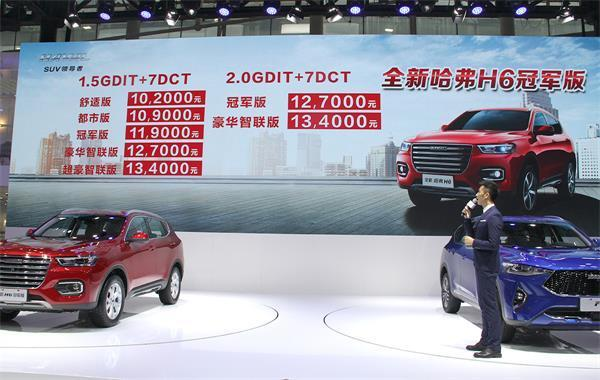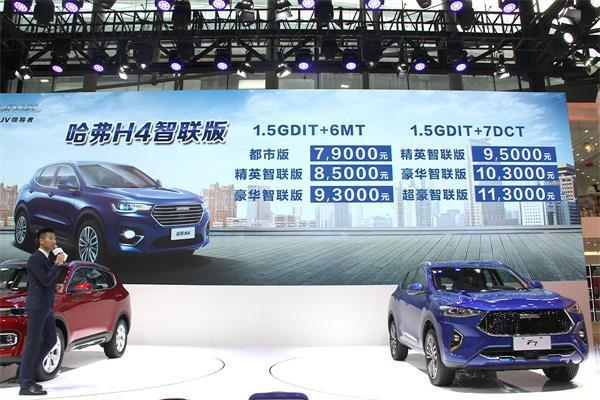两款全新车型均搭载了哈弗智能互联3.0系统，此系统由哈弗品牌与高德、腾讯、博世、夏普等国际一流品质合作伙伴共同打造，具有系统高效稳定、启动快、安全性高、功能丰富等特点。再搭配同级领先的12.3寸超大液晶屏，实现双屏联动，拥有智能语音、智能娱乐、智能远程控制、智能出行四大核心卖点，其中97%语音识别率、2000万在线音乐、10万档在线电台、百兆光纤速度、7*24智能私享管家等功能更是远超同级，使行车更加便利安全，极大地丰富了用户的愉悦驾乘体验。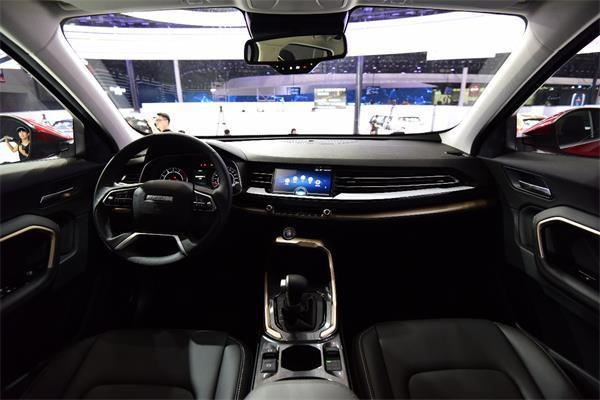焕新品牌形象  F系两款新车交相辉映

本届车展中，哈弗展台的另一大看点则是旗下F系近期相继上市的潮派智联SUV哈弗F5和AI智能网联SUV哈弗F7。作为哈弗品牌旗下专为年轻群体而生的全新细分产品序列，F5、F7两款车型看点十足，瞬间成为镜头下的焦点。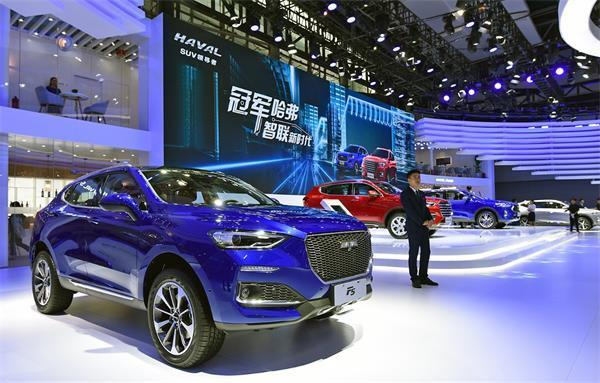其中，哈弗F5作为一款专为90后量身打造的潮派智联SUV，在智能互联、造型美学、驾控体验、智能安全等四大领域均具有强力竞争优势，十分符合现在年轻人的消费心理和用车习惯。在上市首月，哈弗F5便取得6265辆的销量成绩，赢得“开门红”，成为哈弗旗下又一“爆款”车型。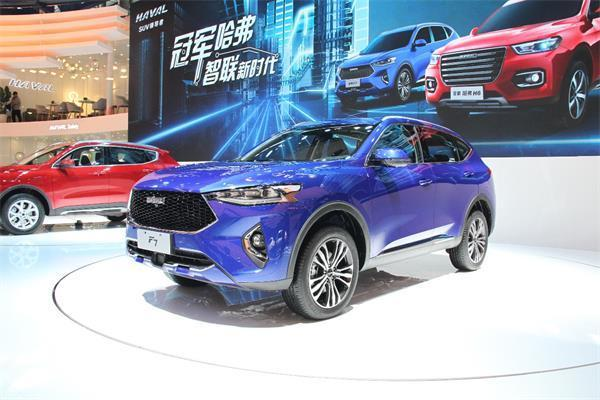不久前刚刚上市的哈弗F7更是在车展中备受瞩目，作为一款追求极致的“AI智能网联SUV”，F7可以用最具颜值、最聪明懂你、最智能安全、最舒适、最全能的SUV“五个最”来形容。无论是时尚潮流的智美造型、强大的AI智能交互功能、丰富豪华的智能科技配置，还是稳健舒适的驾控性能、领先同级的智能安全，都完美展现出其作为中国品牌首款全球车的极致魅力。

随着F系产品的陆续上市，H系产品的升级，哈弗产品矩阵更加丰富，将全面覆盖中国主流SUV市场，而凭借优秀的产品品质、服务口碑和品牌实力，也将极大提升哈弗的市场竞争力。

购置税减半再加现金优惠 热销势不可挡

更值得关注的是，借本次广州车展之际，哈弗诚意推出购置税减半的优惠政策。

具体来说，此次刚刚上市的全新哈弗H6冠军版及哈弗H4智联版一经推出，就能在新车指导价的基础上享受高达50%的购置税补贴；而不久前推出的哈弗F5及哈弗F7也同样参与此次购置税减半活动，最高补贴超过6千元。新车上市之初即如此大幅让利，哈弗可谓诚意十足，再次刷新了SUV价值新标杆。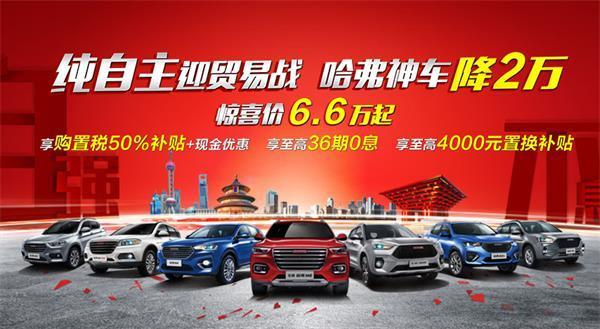除此之外，哈弗热销车型新哈弗H6 Coupe、2018款H6运动版均在购置税减半优惠基础上，另享有现金优惠，优惠额度高达1.5万-2万元；2018款哈弗H2、H2s则享受1.4万-1.7万元不等的现金优惠，哈弗M6更是直接官降达2.4万元，仅售6.6万起。

与此同时，10月份销量数据显示，在车市集体遇冷的背景下，哈弗热销之势依然丝毫不减。在刚过去的金九银十季，哈弗成功实现两连涨，销量逆势上扬，较8月接近翻番，成中国SUV市场绝对王者。随着优惠政策的开展，哈弗必将再迎热销之势。

H、F双线并举  打造全球SUV领导者

2018年，哈弗在继续丰富和优化H系的同时，还在短短时间内发布哈弗全新系列——哈弗F系。至此，哈弗SUV实现H和F双系布局。而两者在定位上明显不同，其中H系打造的是中国最好用的家用SUV，以高性价比、高实用性打动用户。F系则更前卫、更时尚、动感，通过创新设计与智能科技满足用户更多个性化的需求。哈弗H、F系双线并举，在家庭用车和年轻人两大市场共同发力，将覆盖更广泛的消费群体。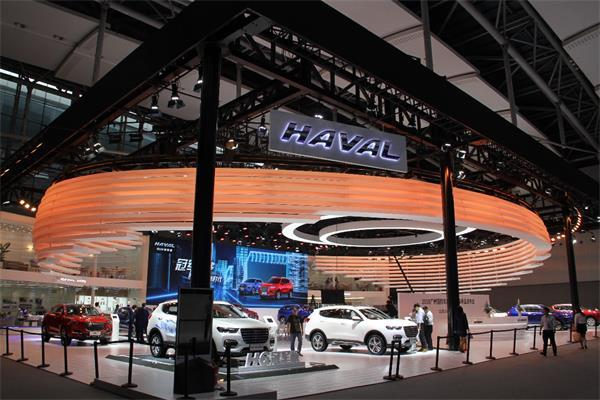除了不断丰富产品阵容，满足更多消费需求外，哈弗一直追求技术上的突破，广泛搭载于全新哈弗H6、H4以及F5、F7等主销车型的“双十佳”动力系统，完全由长城汽车自主研发，其中1.5GDIT发动机，曾荣获“中国心”2017年度十佳发动机称号，7DCT湿式双离合变速器则被誉为“世界十佳变速器”。这套黄金动力组合成功打破了多项国际专利壁垒，完成了哈弗技术的革新。与此同时，哈弗一直致力打造“最安全的SUV”，尤其在智能安全方面，ACC自适应巡航、半自动泊车、360°环视等主动安全配置不断应用在主销车型上，为用户提供了更全面的驾乘安全。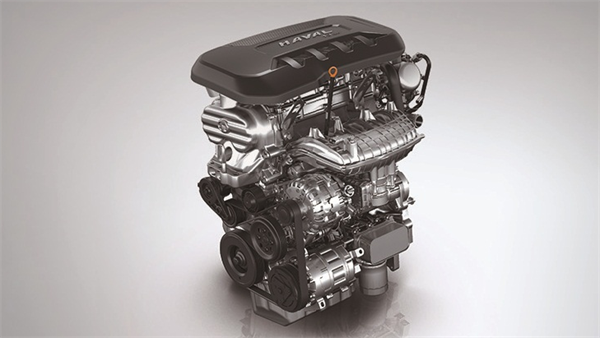智能化作为哈弗品牌的发展新方向，哈弗在未来也将大力发展智能技术，打造高品质、高性价比移动智能终端，继续引领中国自主汽车品牌。哈弗品牌正持续不断的以基础技术、用户思维、个性化服务，三位一体构建全场景化智能服务体系，为用户打造更智能的产品体验，引领行业迈入智能新阶段。发布会上，长城汽车股份有限公司专项副总裁兼哈弗销售公司总经理刘智丰表示：“聚焦SUV品类，是哈弗品牌始终坚守的宏伟战略。从2019年开始，哈弗品牌将以H&F双系和全球化布局为核心开启新征程。全球化竞争是科技含量的比拼，更是科技提前量的PK，哈弗将作为科技的智造者，以创造力定义未来，“研发过度投入”的理念让哈弗在智能科技领域拥有俾倪群雄的‘过度提前量’。”

正是凭借不断创新的理念和产品力的持续升级，在过去的几年，哈弗品牌开创了中国SUV蓝海市场的先河，引领中国SUV从17%-42%的市场增长。迄今为止，哈弗品牌已连续15年获得中国SUV市场销量冠军，旗下哈弗H6更是累计65个月获得SUV销量排行榜冠军，贡献近300万销售业绩，以每分钟销售一辆车的速度创造了中国汽车市场的奇迹，制霸整个SUV市场，无愧“SUV领导者”的称号。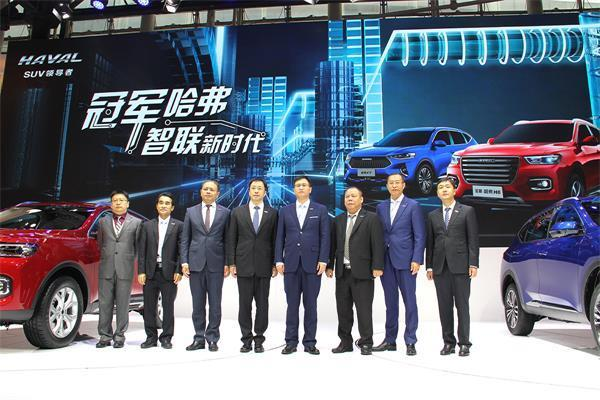如今，哈弗总销量高达近500万辆，成为名副其实的全球销量领先的中国SUV。在未来5年，哈弗品牌将以领导者格局放眼全球，凭借H、F双系战略纵横全球市场，成为在SUV市场中的世界领导者品牌。

##### 相关资讯

|分享

•已阅！握爪
•我手滑为你点赞
•128个赞！
•不明觉厉
•阅后既醉
•有钱！任性
•照片太美，我不敢看
•干货！杠杠的
•高大上
•膜拜中！
•请接受我的膝盖
•猫哥/猫妹么么哒
•神吐槽
•我只笑笑不说话
•我想静静
•窒息
##### 资讯相关车系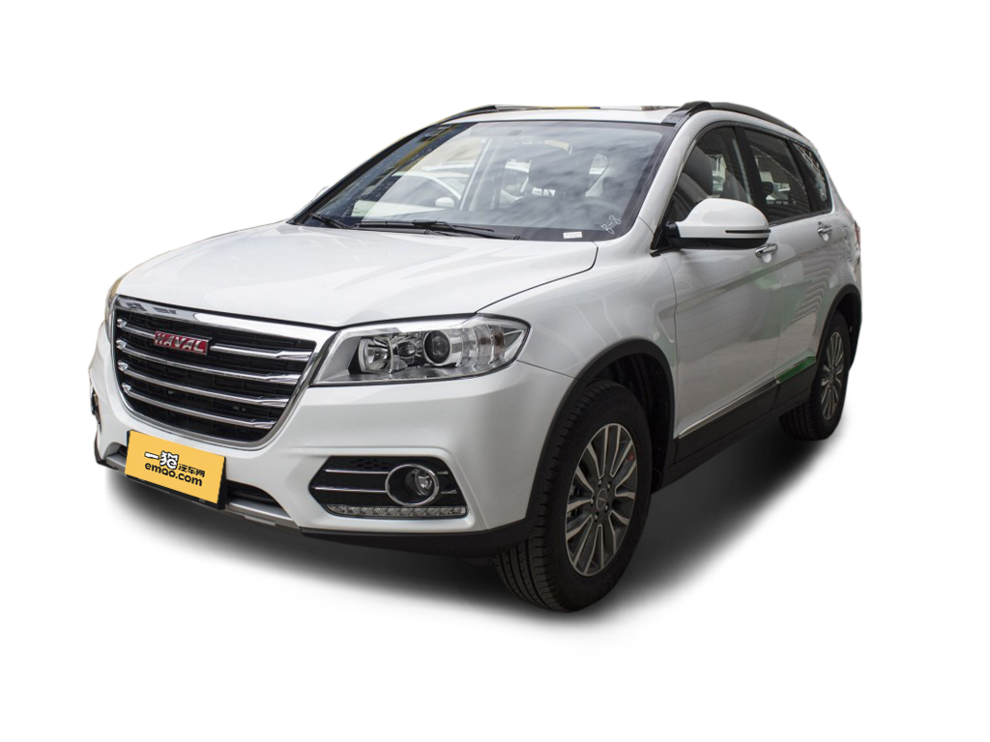##### 热度排行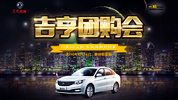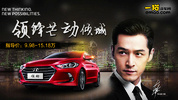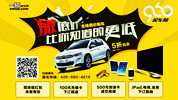• 阿斯顿·马丁
• 奥迪
• 阿尔法罗密欧
• ALPINA
• 爱驰
• AC Schnitzer

• 巴博斯
• 宝骏
• 宝马
• 保时捷
• 北汽制造
• 奔驰
• 奔腾
• 本田
• 比亚迪
• 标致
• 别克
• 宾利
• 布加迪
• 北汽威旺
• 北京
• 北汽绅宝
• 北汽幻速
• 北汽新能源
• 宝沃
• 比速汽车
• 北汽道达
• 北京汽车
• 比德文汽车
• 铂驰
• 北汽昌河

• 昌河
• 长安
• 长城
• 长安商用
• 成功汽车
• 长江EV
• 长安轻车型
• 长安欧尚
• 长安凯程
• 长安跨越
• 车驰汽车

• 大众
• 道奇
• 东风
• 东风风神
• 东风小康
• 东南
• DS
• 东风风行
• 东风风度
• 东风风光
• 电咖
• 大乘汽车
• 大运
• 东风富康

• 法拉利
• 菲亚特
• 丰田
• 福迪
• 福特
• 福田汽车
• 福汽启腾
• 风诺
• 福田

• GMC
• 广汽传祺
• 广汽吉奥
• 观致
• 国金汽车
• 广汽新能源
• 广汽集团
• 国机智骏

• 哈飞
• 海格
• 海马
• 华泰
• 黄海
• 恒天
• 红旗
• 哈弗
• 华颂
• 华凯
• 华泰新能源
• 汉腾汽车
• 华利
• 红星汽车
• HYCAN合创
• 汉龙汽车

• Jeep
• 江淮
• 江铃
• 捷豹
• 金杯
• 九龙
• 吉利汽车
• 金旅
• 金龙
• 江铃集团轻汽
• 江铃集团新能源
• 君马汽车
• 捷途
• 钧天
• 捷达
• 几何汽车

• 开瑞
• 凯迪拉克
• 科尼赛克
• 克莱斯勒
• KTM
• 卡威
• 凯翼
• 康迪
• 康迪电动汽车集团
• 开沃汽车
• 卡升

• 猎豹汽车
• 兰博基尼
• 劳斯莱斯
• 雷克萨斯
• 雷诺
• 理念
• 力帆
• 莲花汽车
• 林肯
• 铃木
• 陆风
• 路虎
• 路特斯
• 领志
• 领克
• 零跑汽车
• Lorinser
• LOCAL MOTORS
• LITE
• 领途汽车
• 理想汽车
• 罗夫哈特
• 力帆汽车

• MG
• MINI
• 马自达
• 玛莎拉蒂
• 迈凯伦
• 摩根
• 名爵
• 迈莎锐
• 迈迈

• 纳智捷
• 哪吒汽车

• 讴歌
• 欧宝
• 欧朗
• 欧拉
• 欧尚汽车

• 帕加尼
• Polestar极星

• 奇瑞
• 启辰
• 起亚
• 前途
• 庆铃汽车
• 骐铃汽车
• 全球鹰
• 乔治·巴顿

• 日产
• 荣威
• 瑞麒汽车
• 如虎
• 瑞驰
• 瑞麒
• 瑞驰新能源
• 容大智造

• 上汽大通
• smart
• 三菱
• 双环
• 双龙
• 斯巴鲁
• 斯柯达
• 萨博
• 思铭
• 赛麟
• SWM斯威汽车
• 上汽MAXUS
• SRM鑫源
• 思皓
• SHELBY
• 上喆

• TESLA
• 泰卡特
• 腾势
• 特斯拉
• 天际汽车

• 威麟
• 威兹曼
• 沃尔沃
• 五菱汽车
• 五十铃
• 潍柴英致
• WEY
• 蔚来
• 威马汽车
• 潍柴汽车

• 现代
• 雪佛兰
• 雪铁龙
• 西雅特
• 新特汽车
• 小鹏汽车
• 新宝骏

• 野马汽车
• 一汽
• 依维柯
• 英菲尼迪
• 永源
• 驭胜
• 云度
• 宇通客车
• 云雀汽车
• 远程汽车
• 一汽凌河

• 中华
• 中兴
• 众泰
• 知豆
• 之诺
• 正道汽车
• A
• B
• C
• D
• E
• F
• G
• H
• I
• J
• K
• L
• M
• N
• O
• P
• Q
• R
• S
• T
• U
• V
• W
• X
• Y
• Z

• 阿斯顿·马丁
• 奥迪
• 阿尔法罗密欧
• ALPINA
• 爱驰
• AC Schnitzer

• 巴博斯
• 宝骏
• 宝马
• 保时捷
• 北汽制造
• 奔驰
• 奔腾
• 本田
• 比亚迪
• 标致
• 别克
• 宾利
• 布加迪
• 北汽威旺
• 北京
• 北汽绅宝
• 北汽幻速
• 北汽新能源
• 宝沃
• 比速汽车
• 北汽道达
• 北京汽车
• 比德文汽车
• 铂驰
• 北汽昌河

• 昌河
• 长安
• 长城
• 长安商用
• 成功汽车
• 长江EV
• 长安轻车型
• 长安欧尚
• 长安凯程
• 长安跨越
• 车驰汽车

• 大众
• 道奇
• 东风
• 东风风神
• 东风小康
• 东南
• DS
• 东风风行
• 东风风度
• 东风风光
• 电咖
• 大乘汽车
• 大运
• 东风富康

• 法拉利
• 菲亚特
• 丰田
• 福迪
• 福特
• 福田汽车
• 福汽启腾
• 风诺
• 福田

• GMC
• 广汽传祺
• 广汽吉奥
• 观致
• 国金汽车
• 广汽新能源
• 广汽集团
• 国机智骏

• 哈飞
• 海格
• 海马
• 华泰
• 黄海
• 恒天
• 红旗
• 哈弗
• 华颂
• 华凯
• 华泰新能源
• 汉腾汽车
• 华利
• 红星汽车
• HYCAN合创
• 汉龙汽车

• Jeep
• 江淮
• 江铃
• 捷豹
• 金杯
• 九龙
• 吉利汽车
• 金旅
• 金龙
• 江铃集团轻汽
• 江铃集团新能源
• 君马汽车
• 捷途
• 钧天
• 捷达
• 几何汽车

• 开瑞
• 凯迪拉克
• 科尼赛克
• 克莱斯勒
• KTM
• 卡威
• 凯翼
• 康迪
• 康迪电动汽车集团
• 开沃汽车
• 卡升

• 猎豹汽车
• 兰博基尼
• 劳斯莱斯
• 雷克萨斯
• 雷诺
• 理念
• 力帆
• 莲花汽车
• 林肯
• 铃木
• 陆风
• 路虎
• 路特斯
• 领志
• 领克
• 零跑汽车
• Lorinser
• LOCAL MOTORS
• LITE
• 领途汽车
• 理想汽车
• 罗夫哈特
• 力帆汽车

• MG
• MINI
• 马自达
• 玛莎拉蒂
• 迈凯伦
• 摩根
• 名爵
• 迈莎锐
• 迈迈

• 纳智捷
• 哪吒汽车

• 讴歌
• 欧宝
• 欧朗
• 欧拉
• 欧尚汽车

• 帕加尼
• Polestar极星

• 奇瑞
• 启辰
• 起亚
• 前途
• 庆铃汽车
• 骐铃汽车
• 全球鹰
• 乔治·巴顿

• 日产
• 荣威
• 瑞麒汽车
• 如虎
• 瑞驰
• 瑞麒
• 瑞驰新能源
• 容大智造

• 上汽大通
• smart
• 三菱
• 双环
• 双龙
• 斯巴鲁
• 斯柯达
• 萨博
• 思铭
• 赛麟
• SWM斯威汽车
• 上汽MAXUS
• SRM鑫源
• 思皓
• SHELBY
• 上喆

• TESLA
• 泰卡特
• 腾势
• 特斯拉
• 天际汽车

• 威麟
• 威兹曼
• 沃尔沃
• 五菱汽车
• 五十铃
• 潍柴英致
• WEY
• 蔚来
• 威马汽车
• 潍柴汽车

• 现代
• 雪佛兰
• 雪铁龙
• 西雅特
• 新特汽车
• 小鹏汽车
• 新宝骏

• 野马汽车
• 一汽
• 依维柯
• 英菲尼迪
• 永源
• 驭胜
• 云度
• 宇通客车
• 云雀汽车
• 远程汽车
• 一汽凌河

• 中华
• 中兴
• 众泰
• 知豆
• 之诺
• 正道汽车
• A
• B
• C
• D
• E
• F
• G
• H
• I
• J
• K
• L
• M
• N
• O
• P
• Q
• R
• S
• T
• U
• V
• W
• X
• Y
• Z

• 阿斯顿·马丁
• 奥迪
• 阿尔法罗密欧
• ALPINA
• 爱驰
• AC Schnitzer

• 巴博斯
• 宝骏
• 宝马
• 保时捷
• 北汽制造
• 奔驰
• 奔腾
• 本田
• 比亚迪
• 标致
• 别克
• 宾利
• 布加迪
• 北汽威旺
• 北京
• 北汽绅宝
• 北汽幻速
• 北汽新能源
• 宝沃
• 比速汽车
• 北汽道达
• 北京汽车
• 比德文汽车
• 铂驰
• 北汽昌河

• 昌河
• 长安
• 长城
• 长安商用
• 成功汽车
• 长江EV
• 长安轻车型
• 长安欧尚
• 长安凯程
• 长安跨越
• 车驰汽车

• 大众
• 道奇
• 东风
• 东风风神
• 东风小康
• 东南
• DS
• 东风风行
• 东风风度
• 东风风光
• 电咖
• 大乘汽车
• 大运
• 东风富康

• 法拉利
• 菲亚特
• 丰田
• 福迪
• 福特
• 福田汽车
• 福汽启腾
• 风诺
• 福田

• GMC
• 广汽传祺
• 广汽吉奥
• 观致
• 国金汽车
• 广汽新能源
• 广汽集团
• 国机智骏

• 哈飞
• 海格
• 海马
• 华泰
• 黄海
• 恒天
• 红旗
• 哈弗
• 华颂
• 华凯
• 华泰新能源
• 汉腾汽车
• 华利
• 红星汽车
• HYCAN合创
• 汉龙汽车

• Jeep
• 江淮
• 江铃
• 捷豹
• 金杯
• 九龙
• 吉利汽车
• 金旅
• 金龙
• 江铃集团轻汽
• 江铃集团新能源
• 君马汽车
• 捷途
• 钧天
• 捷达
• 几何汽车

• 开瑞
• 凯迪拉克
• 科尼赛克
• 克莱斯勒
• KTM
• 卡威
• 凯翼
• 康迪
• 康迪电动汽车集团
• 开沃汽车
• 卡升

• 猎豹汽车
• 兰博基尼
• 劳斯莱斯
• 雷克萨斯
• 雷诺
• 理念
• 力帆
• 莲花汽车
• 林肯
• 铃木
• 陆风
• 路虎
• 路特斯
• 领志
• 领克
• 零跑汽车
• Lorinser
• LOCAL MOTORS
• LITE
• 领途汽车
• 理想汽车
• 罗夫哈特
• 力帆汽车

• MG
• MINI
• 马自达
• 玛莎拉蒂
• 迈凯伦
• 摩根
• 名爵
• 迈莎锐
• 迈迈

• 纳智捷
• 哪吒汽车

• 讴歌
• 欧宝
• 欧朗
• 欧拉
• 欧尚汽车

• 帕加尼
• Polestar极星

• 奇瑞
• 启辰
• 起亚
• 前途
• 庆铃汽车
• 骐铃汽车
• 全球鹰
• 乔治·巴顿

• 日产
• 荣威
• 瑞麒汽车
• 如虎
• 瑞驰
• 瑞麒
• 瑞驰新能源
• 容大智造

• 上汽大通
• smart
• 三菱
• 双环
• 双龙
• 斯巴鲁
• 斯柯达
• 萨博
• 思铭
• 赛麟
• SWM斯威汽车
• 上汽MAXUS
• SRM鑫源
• 思皓
• SHELBY
• 上喆

• TESLA
• 泰卡特
• 腾势
• 特斯拉
• 天际汽车

• 威麟
• 威兹曼
• 沃尔沃
• 五菱汽车
• 五十铃
• 潍柴英致
• WEY
• 蔚来
• 威马汽车
• 潍柴汽车

• 现代
• 雪佛兰
• 雪铁龙
• 西雅特
• 新特汽车
• 小鹏汽车
• 新宝骏

• 野马汽车
• 一汽
• 依维柯
• 英菲尼迪
• 永源
• 驭胜
• 云度
• 宇通客车
• 云雀汽车
• 远程汽车
• 一汽凌河

• 中华
• 中兴
• 众泰
• 知豆
• 之诺
• 正道汽车
• A
• B
• C
• D
• E
• F
• G
• H
• I
• J
• K
• L
• M
• N
• O
• P
• Q
• R
• S
• T
• U
• V
• W
• X
• Y
• Z